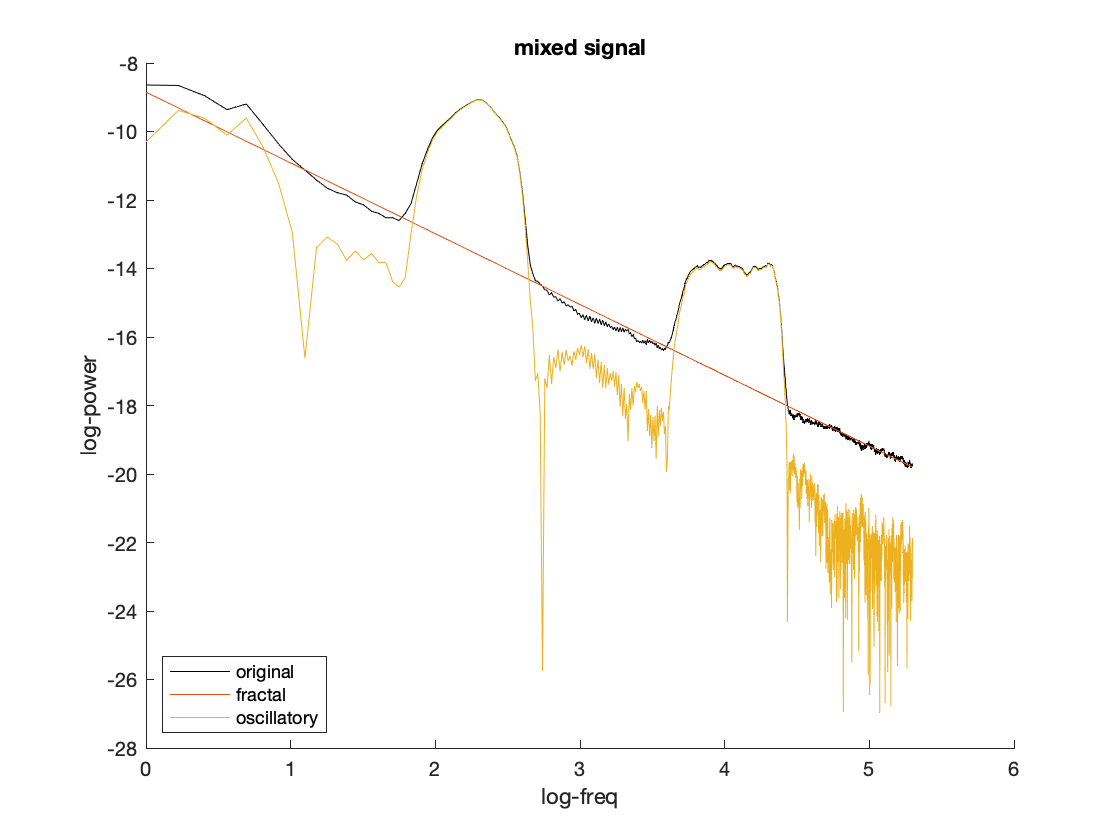Tags: example fooof

# Fitting oscillations and one-over-F (FOOOF)

FOOOF allows distinguishing rhythmic activity from concurrent power-spectral 1/f modulations. The implementation in FieldTrip is using code from the Brainstorm toolbox. The Brainstorm website has a tutorial that provides some more details, there is a GitHub project with more tutorials and documentation, and the FOOOF reference paper explains and demonstrates it.

The following code shows how to extract spectral features from simulated data.

``````% simulate data
F = 1; % weight of (F)ractal components of the simulated data
O = 1; % weight of (O)scillatory components of the simulated data
t = (1:60000)/1000; % time axis
for rpt = 1:1
% use a simple method to make pink noise that does not rely on the digital signal processing toolbox
fn = cumsum(randn(1,length(t)));
fn = fn./max(abs(fn));

sgn10 = ft_preproc_bandpassfilter(randn(1,length(t)),1000,[8 12],[],'firws');
sgn10 = 0.15.*sgn10./max(abs(sgn10));

sgn60 = ft_preproc_bandpassfilter(randn(1,length(t)),1000,[40 80],[],'firws');
sgn60 = 0.05.*sgn60./max(abs(sgn60));

% add a 10 Hz and 60 Hz oscillation
data.trial{1,rpt} = F * fn + O * sgn10 + O * sgn60;
data.time{1,rpt}  = t;
data.label{1}     = 'chan';
data.trialinfo(rpt,1) = rpt;
end

% chunk into 2-second segments
cfg               = [];
cfg.length        = 2;
cfg.overlap       = 0.5;
data              = ft_redefinetrial(cfg, data);

% compute the fractal and original spectra
cfg               = [];
cfg.foilim        = [1 200];
cfg.tapsmofrq     = 2;
cfg.method        = 'mtmfft';
cfg.output        = 'fooof_aperiodic';
fractal = ft_freqanalysis(cfg, data);
cfg.output        = 'pow';
original = ft_freqanalysis(cfg, data);

% subtract the fractal component from the power spectrum
cfg               = [];
cfg.parameter     = 'powspctrm';
cfg.operation     = 'x2-x1';
oscillatory = ft_math(cfg, fractal, original);

% display the spectra on a log-log scale
figure();
hold on;
plot(log(original.freq), log(original.powspctrm),'k');
plot(log(fractal.freq), log(fractal.powspctrm));
plot(log(fractal.freq), log(oscillatory.powspctrm));
xlabel('log-freq'); ylabel('log-power');
legend({'original','fractal','oscillatory'},'location','southwest');
if F~=0 && O==0
title('pure fractal signal');
elseif F==0 && O~=0
title('pure oscillatory signal');
elseif F~=0 && O~=0
title('mixed signal');
end
``````